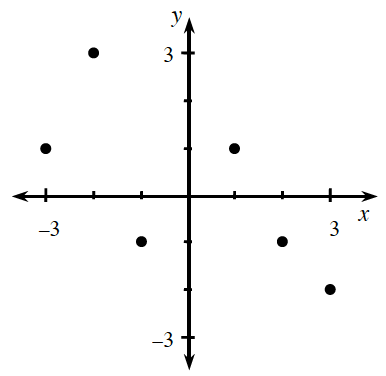Home > ACC7 > Chapter cc31 > Lesson cc31.1.2 > Problem1-16

1-16.

Use the graph at right to answer the following questions about quadrants and coordinates of points. Read the Math Notes box in this lesson if you need to review these concepts. Homework Help ✎

1. What are the coordinates of the two points in Quadrant II?

Quadrant I is in the top right of a graph.

Match the number on the $x$-coordinate with the number on the $y$-coordinate of the two points in Quadrant II.

$(−3,1)$ and $(−2,3)$

2. What are the coordinates of the two points in Quadrant IV?

Part (b) uses the same steps as part (a). Just use Quadrant IV instead of Quadrant II.

$(2,−1)$ and $(3,−2)$